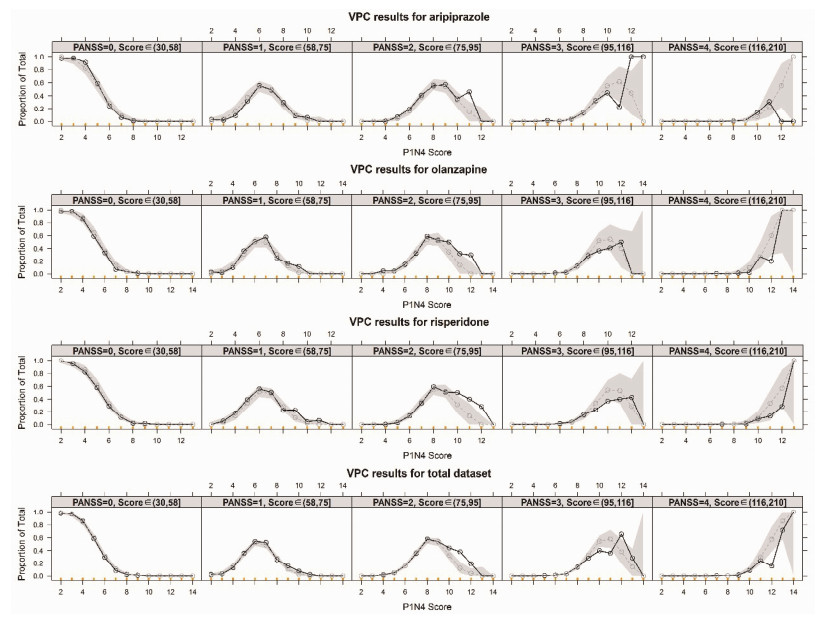﻿ 应用累积比数Logit模型对PANSS评分表衍生调查问卷的初步分析
 药学学报2016, Vol. 51Issue (10): 1572-1577PDF

1. 北京大学天然药物及仿生药物国家重点实验室, 北京 100191 ;
2. 北京大学医学部药学院药剂学系, 北京 100191 ;
3. 首都医科大学附属北京安定医院, 北京 100088 ;
4. 北京脑重大疾病研究院精神分裂症研究所, 北京 100088

Using the cumulative odds Logit model to explore the application of the questionnaire derived from PANSS
JI Shuang-min1,2, LI An-ning3, DONG Fang3, LI Liang1,2, ZHOU Tian-yan1,2, WANG Chuan-yue3,4, LU Wei1,2,41. State Key Laboratory of Natural and Biomimetic Drugs, Peking University, Beijing 100191, China ;
2. School of Pharmaceutical Sciences, Peking University, Beijing 100191, China ;
3. Beijing Anding Hospital, Capital Medical University, Beijing 100088, China ;
4. Center of Schizophrenia, Beijing Institute for Brain Disorders, Beijing 100088, China
Abstract: Currently, aripiprazole, olanzapine and risperidone are three anti-psychiatry agents commonly used in the treatment of schizophrenia. Although the efficacy of these drugs is good, schizophrenia cannot be completely cured yet. Patients need long-term medication. The family members of patients may play a key role to understand the disease status of patients after patient discharge from hospital. PANSS is a commonly used scale in the clinic to evaluate the disease status and drug effects of anti-psychiatry agents. It was professionally written, and is not user friendly to amateurs. In the previous study, we developed a questionnaire for patient's family members to monitor the disease status. In this study, we explored the correlations between the results of questionnaire and 5 kinds of disease state corresponding to different PANSS score interval using the cumulative odds Logit model. The final results show that the model had relatively good prediction ability for aripiprazole, olanzapine and risperidone, suggesting that the questionnaire has an extensive prospect of clinical applications.
Key words: cumulative odds Logit model     positive and negative syndrome scale     schizophrenia     clinical applicationTable 1 The definition and description of 5 different disease state corresponding to different PANSS score intervals

 ${{P}_{j}}={{P}_{\text{TV}}}+{{\eta }_{j}}$ (1)
 ${{P}_{j}}={{P}_{\text{TV}}}\times {{\eta }_{j}}$ (2)
 ${{P}_{j}}={{P}_{\text{TV}}}\times \exp \left( {{\eta }_{j}} \right)$ (3)Table 2 Baseline clinical characteristics and demographic information of patients included in this study
2 建模与评价

 $\text{Logit}\left( {{P}_{\text{PANSS}\ge i}} \right)=\ln \left( \frac{{{P}_{\text{PANSS}\ge i}}}{1-{{P}_{\text{PANSS}\ge i}}} \right)$ (4)

 ${{P}_{\text{PANSS}\ge i}}=\frac{{{e}^{\text{Logit}\left( {{P}_{\text{PANSS}\ge i}} \right)}}}{1+{{e}^{\text{Logit}\left( {{P}_{\text{PANSS}\ge i}} \right)}}}$ (5)

 ${{P}_{\text{PANSS}\ge i}}\text{=}{{P}_{\text{PANSS}\ge i}}-{{P}_{\text{PANSS}\ge i+1}}$ (6)
 $\text{Logit}\left( {{P}_{\text{PANSS}\ge i}} \right)\text{=}f\left( \theta ,\operatorname{cov},\eta \left| PIN4 \right. \right)$ (7)

 $\text{Logit}\left( {{P}_{\text{PANSS}\ge 1}} \right)+{{\theta }_{1}}+{{\theta }_{5}}\times \text{PIN}4+\eta$ (8)
 $\text{Logit}\left( {{P}_{\text{PANSS}\ge 2}} \right)+{{\theta }_{1}}+{{\theta }_{2}}+{{\theta }_{5}}\times \text{PIN}4+\eta$ (9)
 $\text{Logit}\left( {{P}_{\text{PANSS}\ge 3}} \right)+{{\theta }_{1}}+{{\theta }_{2}}+{{\theta }_{3}}+{{\theta }_{5}}\times \text{PIN}4+\eta$ (10)
 $\text{Logit}\left( {{P}_{\text{PANSS}\ge 4}} \right)+{{\theta }_{1}}+{{\theta }_{2}}+{{\theta }_{3}}+{{\theta }_{4}}+{{\theta }_{5}}\times \text{PIN}4+\eta$ (11)

 ${{P}_{\text{PANSS}=0}}=1-\frac{{{e}^{\text{Logit}\left( {{P}_{\text{PANSS}\ge 1}} \right)}}}{1+{{e}^{\text{Logit}\left( {{P}_{\text{PANSS}\ge 1}} \right)}}}$ (12)
 ${{P}_{\text{PANSS}=i}}=\frac{{{e}^{\text{Logit}\left( {{P}_{\text{PANSS}\ge i}} \right)}}}{1+{{e}^{\text{Logit}\left( {{P}_{\text{PANSS}\ge i}} \right)}}}-\frac{{{e}^{\text{Logit}\left( {{P}_{\text{PANSS}\ge i+1}} \right)}}}{1+{{e}^{\text{Logit}\left( {{P}_{\text{PANSS}\ge i+1}} \right)}}}$ (13)
 ${{P}_{\text{PANSS}=4}}=\frac{{{e}^{\text{Logit}\left( {{P}_{\text{PANSS}\ge 4}} \right)}}}{1+{{e}^{\text{Logit}\left( {{P}_{\text{PANSS}\ge 4}} \right)}}}$ (14)
3 模型参数的估算Table 3 Population parameters of final proportional odds model for aripiprazole, olanzapine, risperidone and the total datasetFigure 1 Visual predictive check plot for the model of aripiprazole, olanzapine, risperidone and the total dataset. The black and grey dots represented the observed and predicted value, respectively. The black solid line referred to the connections of observations while the grey dashed line referred to predicted median line. And the grey shadow represented the predicted 95% confidence interval

  Picchioni MM, Murray RM. Schizophrenia[J]. BMJ , 2007, 335 :91–95. DOI:10.1136/bmj.39227.616447.BE  Hor K, Taylor M. Suicide and schizophrenia:a systematic review of rates and risk factors[J]. J Psychopharmacol , 2010, 24 :81–90. DOI:10.1177/1359786810385490  Schizophrenia[EB/OL]. http://www.mentalhealth.com/home/dx/schizophrenia_advanced.html.  Magliano L, Fiorillo A. Family burden in long-term diseases:a comparative study in schizophrenia vs physical disorders[J]. Soc Sci Med , 2005, 61 :313–322. DOI:10.1016/j.socscimed.2004.11.064  Lindenmayer JP, Harvey PD, Khan A, et al. Schizophrenia:measurements of psychopathology[J]. Psychiatr Clin North Am , 2007, 30 :339–363. DOI:10.1016/j.psc.2007.04.005  Newman JM, Turnbull A, Berman BA, et al. Impact of traumatic and violent victimization experiences in individuals with schizophrenia and schizoaffective disorder[J]. J Nerv Ment Dis , 2010, 198 :708–714. DOI:10.1097/NMD.0b013e3181f49bf1  Leucht S, Corves C, Arbter D, et al. Second-generation versus first-generation antipsychotic drugs for schizophrenia:a meta-analysis[J]. Lancet , 2009, 373 :31–41. DOI:10.1016/S0140-6736(08)61764-X  Jenkins JH, Carpenter-Song E. The new paradigm of recovery from schizophrenia:cultural conundrums of improvement without cure[J]. Cult Med Psychiatry , 2005, 29 :379–413. DOI:10.1007/s11013-006-9000-8  Caqueo-Urizar A, Rus-Calafell M, Urzua A, et al. The role of family therapy in the management of schizophrenia:challenges and solutions[J]. Neuropsychiatr Dis Treat , 2015, 11 :145–151.  Kay SR, Fiszbein A, Opler LA. The positive and negative syndrome scale (PANSS) for schizophrenia[J]. Schizophr Bull , 1987, 13 :261–276. DOI:10.1093/schbul/13.2.261  Kay SR, Opler LA, Lindenmayer JP. The positive and negative syndrome scale (PANSS):rationale and standardization[J]. Br J Psychiatry , 1989, Suppl :59–67.  Li WS, Zhao Y, Fang W. The effect of group psychotherapy on improving the symptom of middle-aged or youth schizophrenia patients[J]. China J Health Psychol (中国健康心理学杂志) , 2014, 22 :331–333.  Ji SM, Li AN, Wu KH, et al. Brief questionnaire derived from PANSS using a general probability model to assess and monitor the clinical features of schizophrenia[J]. Pharmacopsychiatry , 2016, 49 :117–123. DOI:10.1055/s-00000054  Yue WH, Wang HF, Sun LD, et al. Genome-wide association study identifies a susceptibility locus for schizophrenia in Han Chinese at 11p11.2[J]. Nat Genet , 2011, 43 :1228–1231. DOI:10.1038/ng.979  Andreasen NC, Carpenter WT Jr., Kane JM, et al. Remission in schizophrenia:proposed criteria and rationale for consensus[J]. Am J Psychiatry , 2005, 162 :441–449. DOI:10.1176/appi.ajp.162.3.441  Leucht S, Kane JM, Kissling W, et al. What does the PANSS mean?[J]. Schizophr Res , 2005, 79 :231–238. DOI:10.1016/j.schres.2005.04.008  Opler MG, Yang LH, Caleo S, et al. Statistical validation of the criteria for symptom remission in schizophrenia:preliminary findings[J]. BMC Psychiatry , 2007, 7 :35. DOI:10.1186/1471-244X-7-35  Ren YP, Deng CH, Wang XP, et al. Comparison study of model evaluation methods:normalized prediction distribution errors vs visual predictive check[J]. Acta Pharm Sin (药学学报) , 2011, 46 :1123–1131.  Beal SL, Sheiner LB, Boeckmann AJ. NONMEM User's Guide-Part I-VⅡ[M]. Ellicott City, MD: Icon Development Solutions, 1989-2006 .